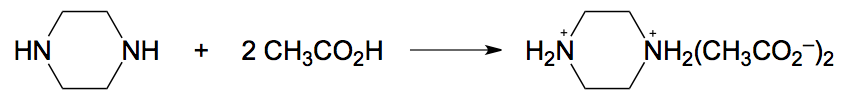Clutch Prep is now a part of Pearson
Ch.16 - Electroanalytical TechniquesWorksheetSee all chapters

# Gravimetric Analysis

See all sections
Sections
Fundamentals of Electrolysis
Gravimetric Analysis
Coulometry
Voltammetry

In Gravimetric Analysis the mass of a product in a chemical reaction is used to calculate the amount of the original analyte.

###### Introduction to Gravimetric Analysis

Example #1:

A 25.00 mL solution containing Br was treated with excess PbSO4 to precipitate 0.7550 g of PbBr2. What was the molarity of the Br in the unknown?

PbSO4 (s)   +   2 Br (aq) → PbBr2 (s)   +SO42– (aq)

###### Expert Q&A

Ask unlimited questions and get expert help right away.

Example #2: The Fe in a 1.1530 g sample of iron ore is precipitated as Fe2O3 x H2O by the addition of NH3. The residue is ignited at high temperatures to give 0.6310 g of pure Fe2O3. Calculate the weight percent of Fe in the ore.

2 Fe3+ (aq) +  6 OH (aq) → Fe2O3 * x H2O

Fe2OxH2O +  heat  → Fe2O3 +  6 x H2O

###### Expert Q&A

Ask unlimited questions and get expert help right away.

###### Electrogravimetric Analysis Calculations

Example #3: The reaction between piperazine and acetic acid creates an adduct product known as piperazine diacetate.

A 7.50 g sample of impure piperazine contained 83.01% piperazine. How many grams of piperazine diacetate would be formed in the process?

###### Expert Q&A

Ask unlimited questions and get expert help right away.Example #4: The amount of iron within an ore sample was determined by an oxidation-reduction titration using potassium permanganate, KMnO­4, as the titrant. A 0.5600 g sample of the ore was placed into acid and the newly freed Fe3+ was then reduced to Fe2+. The titration of this solution required 39.82 mL of 0.0315 M KMnO­4 to reach the end-point. Determine the mass percent of Fe2O3 in the sample.

MnO4   +5 Fe2+   + 8 H+ → Mn2+  +  5 Fe3+  +  4 H2O

###### Expert Q&A

Ask unlimited questions and get expert help right away.

###### Expert Q&A

Ask unlimited questions and get expert help right away.